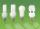Bureau of Labor

Bureau of Labor is a state institution that provides mike and the rest for their so-called clients. The mission of the Bureau of Labor is spend taxpayer money to provide relaxation and benefits to those who do not want to work. Popularly speaking sense the work of the Bureau of Labor is to create the largest number of unemployed. This helps him massively Labour Code, trade unions, minimum wage and other regulations that were not here.

Back to mathematics. Probability that Bureau of Labor "finds" unemployed job is 1.7%. Probability that a job seeker finds job as active person is 93%.

Calculate how many times the probability of finding a job more his own efforts than through Bureau of Labor.

Result

x =  54.7

Solution:Leave us a comment of this math problem and its solution (i.e. if it is still somewhat unclear...):Be the first to comment!To solve this verbal math problem are needed these knowledge from mathematics:

Need help calculate sum, simplify or multiply fractions? Try our fraction calculator. Our percentage calculator will help you quickly calculate various typical tasks with percentages. Would you like to compute count of combinations?

Next similar math problems:

1. BulbsIn the box are 6 bulbs with power 75 W, 14 bulbs with power 40 W and 15 with 60 W. Calculate probability that a randomly selected bulb is:
2. WithdrawalIf I withdrew 2/5 of my total savings and spent 7/10 of that amount. What fraction do I have in left in my savings?
3. TeacherTeacher Rem bought 360 pieces of cupcakes for the outreach program of their school. 5/9 of the cupcakes were chocolate flavor and 1/4 wete pandan flavor and the rest were a vanilla flavor. How much more pandan flavor cupcakes than vanilla flavor?
4. Passenger boatTwo-fifths of the passengers in the passenger boat were boys. 1/3 of them were girls and the rest were adult. If there were 60 passengers in the boat, how many more boys than adult were there?
5. In fractionsAn ant climbs 2/5 of the pole on the first hour and climbs 1/4 of the pole on the next hour. What part of the pole does the ant climb in two hours?
6. Pizza 4Marcus ate half pizza on monday night. He than ate one third of the remaining pizza on Tuesday. Which of the following expressions show how much pizza marcus ate in total?
7. Product of two fractionsProduct of two fractions is 9 3/5 . If one of the fraction is 9 3/7. Find the other fraction.
8. IronIron ore contains 57% iron. How much ore is needed to produce 20 tons of iron?
9. Fraction and a decimalWrite as a fraction and a decimal. One and two plus three and five hundredths
10. PointsGryffindor won 437 points. How many points obtained by each of the faculties if they were split at a ratio of 5: 7: 3: 4?
11. Cupcakes 2Susi has 25 cupcakes. She gives 4/5. How much does she have left?
12. ClassIn 7.C clss are 10 girls and 20 boys. Yesterday was missing 20% of girls and 50% boys. What percentage of students missing?
13. ZdeněkZdeněk picked up 15 l of water from a 100-liter full-water barrel. Write a fraction of what part of Zdeněk's water he picked.
14. Write 2Write 791 thousandths as fraction in expanded form.
15. Ratio 11Simplify this ratio 10 : 1/4
16. Fraction to decimalWrite the fraction 3/22 as a decimal.
17. Lengths of the poolMiguel swam 6 lengths of the pool. Mat swam 3 times as far as Miguel. Lionel swam 1/3 as far as Miguel. How many lengths did mat swim?